# Solution assignment 10 Quadratic equations (abc-formula)

### Assignment 10

For which value(s) of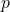does the equation::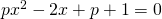have no solutions.

### Solution

When we want to know how many solutions a quadratic equation has, we look at the discriminant: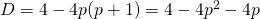The equation may not have solutions, thus the discriminant has to be negative: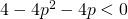or: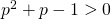We know that the graph of the function: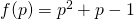is an 'opens up' parabola which intersects the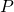-axis in the points: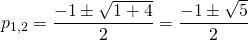This parabola is positive if: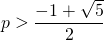or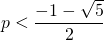0